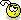## Recommended Posts

Okies, after studying the help file i managed to do this:

```\$cs = IniRead("C:\x\y\Desktop\variable.ini", "Variable", "MouseClick", "NotFound")
Sleep(500)
If \$cs = 1 Then
MouseClick("Left", 180, 145, 1, 1)
MouseClick("Left", 180, 145, 1, 1)
EndIf
If \$cs = 2 Then
MouseClick("Left", 466, 146, 1, 1)
MouseClick("Left", 466, 146, 1, 1)
EndIf
If \$cs = 3 Then
MouseClick("Left", 179, 240, 1, 1)
MouseClick("Left", 179, 240, 1, 1)
EndIf
If \$cs = 4 Then
MouseClick("Left", 459, 246, 1, 1)
MouseClick("Left", 459, 246, 1, 1)
EndIf
If \$cs = 5 Then
MouseClick("Left", 172, 336, 1, 1)
MouseClick("Left", 172, 336, 1, 1)
EndIf
If \$cs = 6 Then
MouseClick("Left", 453, 332, 1, 1)
MouseClick("Left", 453, 332, 1, 1)
EndIf
If \$cs = 7 Then
MouseClick("Left", 177, 431, 1, 1)
MouseClick("Left", 177, 431, 1, 1)
EndIf
If \$cs = 8 Then
MouseClick("Left", 454, 429, 1, 1)
MouseClick("Left", 454, 429, 1, 1)
EndIf```

Ini

```[variable]
MouseClick=1```

Dont laugh. Im kinda new to this and im proud of that. Now then, my first of the two questions: Can this code be shortened anyway in length? If not no worries. Secondly...

Assuming that the .exe file and the .ini file are in the same folder, is there a way to make it detect this automatically? So that the IniRead = (C:/x/x/x.ini) can automatically be detected, if that makes any sense?Thanks,

Moja

Edited by Moja

##### Share on other sites

Something like this?

```\$CS = IniRead(@ScriptDir & "\variable.ini", "Variable", "MouseClick", "NotFound")
Sleep(500)
Select
Case \$CS = 1
MouseClick("Left", 180, 145, 2, 1)
Case \$CS = 2
MouseClick("Left", 466, 146, 2, 1)
Case \$CS = 3
MouseClick("Left", 179, 240, 2, 1)
Case \$CS = 4
MouseClick("Left", 459, 246, 2, 1)
Case \$CS = 5
MouseClick("Left", 172, 336, 2, 1)
Case \$CS = 6
MouseClick("Left", 453, 332, 2, 1)
Case \$CS = 7
MouseClick("Left", 177, 431, 2, 1)
Case \$CS = 8
MouseClick("Left", 454, 429, 2, 1)
EndSelect```
Edited by ezzetabi

##### Share on other sites

Fantastic! Thanks, now I have learnt about the Select, Case, EndSelect function, which will be nice, really appreciate it, thanks!

Moja

## Create an account

Register a new account

• ### Recently Browsing   0 members

×

• Wiki

• Back

• #### Beta

• Git
• FAQ
• Our Picks
×
• Create New...